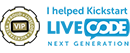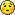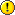Interpolate

Anything beyond the basics in using the LiveCode language. Share your handlers, functions and magic here.

Moderators: Klaus, FourthWorld, heatherlaine, robinmiller, kevinmiller

Fermin
Posts: 77
Joined: Fri Jun 05, 2015 10:44 pm

Interpolate

Hello. Could someone please help me with a method of interpolating values? At first I thought it was simple, but I've been doing it for quite some time and I can't find the solution.
It's about calculating intermediate values - produced by a power - between two proposed values.

I get it to work in a simple sequence (the script I include) but I need those values to be adjusted proportionally to some proposed limit values, for example, from 605 to 730, that is, to calculate (following the example) the eight intermediate values between the first (605) and the last (730).

I hope I've been able to explain what I want. Thank you very much in advance and Happy 2019.

Fermin

Translated with DeepL

--------------------------------

Code: Select all

on mouseup
put 10 into nSteps -- Number of Steps
put 1.8 into ePower -- Increment Manager
--
put 605 into vFirst -- Value of the first step (to use in the missing code)
put 730 into vLast -- Value of the last step (to use in the missing code)
-----
repeat with a = 1 to nSteps
put a^ePower into v1
put a && "___" && v1 into line a of ccc
end repeat
put ccc
end mouseup

[-hh]
VIP Livecode Opensource BackerPosts: 2122
Joined: Thu Feb 28, 2013 11:52 pm
Location: Göttingen, DE

Re: Interpolate

Fermin wrote:I hope I've been able to explain what I want.
No, not really. But here is one guess:

The start value v1=605 and the end value v2=730 are fixed params.
The exponent xp=1.8 and the num of steps n=10 are fixed params.

Now you want the 11 values a1,a2,...,a11, increasing in 10 equal steps such that a1^1.8=605 and a11^1.8=730.

These values and their function values are given by (returns 11 lines with value -> function value)

Code: Select all

on mouseUp
local v1=605, v2=730, xp=1.8, n=10
put exp(ln(v1)/xp) into a1 --# that is a1^xp=v1
put exp(ln(v2)/xp) into a2 --# that is a2^xp=v2
put (a2-a1)/n into d
repeat with t=a1 to a2 step d
## value -> function value
put cr& t &" -> "& t^xp after s
end repeat
put char 2 to -1 of s into fld "OUT"
end mouseUp
With your example set of parameters the result is as follows.

Code: Select all

35.109096 -> 605
35.495227 -> 617.029505
35.881358 -> 629.164156
36.267489 -> 641.403729
36.653620 -> 653.747996
37.039751 -> 666.196738
37.425882 -> 678.749736
37.812013 -> 691.406773
38.198144 -> 704.167636
38.584274 -> 717.032114
38.970405 -> 730
HTH. All the best for 2019! (Also to everybody who reads this.)
shiftLock happens

Fermin
Posts: 77
Joined: Fri Jun 05, 2015 10:44 pm

Re: Interpolate

Thank you very much for your reply. But I think I've raised the question wrong and it's a lot more complex. In fact, now I don't know which function is the right one to get what I'm looking for.

What I want to achieve are NOT equal values between steps but 'curves' of acceleration or deceleration like those used between keyframes in video editing programs (After Effects, Final Cut etc). That's why I've used the powers as a value generator but now I'm not sure it's the right method. Maybe I should use logarithms or trigonometric functions.

I have simulated some examples of the results I am looking for

Slow ascent
1,605
2,606
3,610
4,619
5,622
6,627
7,637
8,655
9,684
10,730

Rapid ascent
1,605
2,638
3,658
4,674
5,687
6,697
7,707
8,715
9,723
10,730

Like I said, I think it's a lot harder than it looks. So far I'm using a little 'homemade' methods that get me out of trouble but, of course, they're not very good.

Anyway, the code you sent me has given me some good ideas. Thanks again.

[-hh]
VIP Livecode Opensource BackerPosts: 2122
Joined: Thu Feb 28, 2013 11:52 pm
Location: Göttingen, DE

Re: Interpolate

[This not complex.]

Given a start value v1, an end value v2, a number of steps n.
You want a power function that increases slower or faster than linear, such that the values are ascending and in between v1 and v2.

The easiest way to do that is to choose a power p and transform the function x^p from the interval [0,1] to the interval [v1,v2].
• p=1 is linear.
That is you'll get n equal steps between v1 and v2.
• p < 1, say p between 0.5 and 1, increases faster than linear (rapid ascent),
the faster the more distant from 1.
• p > 1, say p between 1 and 3, increases slower than linear (slow ascent),
the slower the more distant from 1.
Obviously you need integers as output, so you could script:

Code: Select all

on mouseUp
local v1=605, v2=730, p=2, n=10
-- put the thumbpos of sb "power" into p
-- put the thumbpos of sb "steps" into n
put (v2-v1)/(n^p-1) into b
repeat with i=1 to n
put cr & i,round(v1+b*(i^p-1)) after s
end repeat
put char 2 to -1 of s into fld "OUT"
end mouseUp
shiftLock happens

Fermin
Posts: 77
Joined: Fri Jun 05, 2015 10:44 pm

Re: Interpolate

What a great Christmas present.I'm doing the first tests with your new code and I think it's exactly what I was looking for.
I'm going to adapt it and integrate it into an application in LiveCode to generate routes for Google Earth. I am very grateful to you, [-hh].
Fermin

[-hh]
VIP Livecode Opensource BackerPosts: 2122
Joined: Thu Feb 28, 2013 11:52 pm
Location: Göttingen, DE

Re: Interpolate

Fermin,
I remember from other threads some time ago that you are an video expert. Would be nice to see (a preview/test version of) your app in livecodeshare.

Hermann
shiftLock happens

capellan
Posts: 610
Joined: Wed Aug 15, 2007 11:09 pm
Contact:

Re: Interpolate

golife
Posts: 25
Joined: Fri Apr 02, 2010 12:10 pm

Re: Interpolate

Thanks a lot for the answers to the interpolate question.

I have the questions the other way around depending upon width and height of an object, the price for such object changes in a non-linear form. How to find a function that would approximate the price values found?

I believe this question has to do with "interpolation" of values and that is why I am posting it here.

Example: The following list values are known:
x-min = 100, x-max = 600
y-min = 100, y-max = 600
USD-max = 900, USD-min = 30

x = 100, y = 100, price = USD 30
x = 200, y = 300, price = USD 120
x = 300, y = 400, price = USD 250
x = 400, y = 500, price = USD 600
x = 600, y = 600, price = USD 900

Now we would like to enter any x or y value within the range of values to come up with a price based on a function calculating an approximate value.

I understand that the more values are known the closer such function to what we are looking for.
It is assumed that some logarithmic function can be defined and values are not just arbitrary.

Is there a solution for LiveCode that would allow us programmatically to be close to an ideal dollar value?

[-hh]
VIP Livecode Opensource BackerPosts: 2122
Joined: Thu Feb 28, 2013 11:52 pm
Location: Göttingen, DE

Re: Interpolate

You have prices depending on size = x*y (width*height).

So your prices are, reading x and y as centimeters, for the sizes in meters:
p(1)=30
p(6)=120
p(12)=250
p(20)=600
p(36)=900

That is you have points
(1,30)
(6,20)
(12,250)
(20,600)
(36,900)

Now you could compute, depending on your "non-linear model", a polynomial or log-linear regression curve to "fit" these points.

This is simple math, not at all difficult, doable in LC, but tedious to script.
shiftLock happens

Fermin
Posts: 77
Joined: Fri Jun 05, 2015 10:44 pm

Re: Interpolate

Fermin,
I remember from other threads some time ago that you are an video expert. Would be nice to see (a preview/test version of) your app in livecodeshare.

Hermann
---
No problem, Hermann... but I don't think my apps can serve anyone.
The applications I make are tools to act on specific parts of my projects. They are developed with no intention of publishing, so their user interfaces tend to be a dreadful patchwork of controls that I continually modify; in fact, there are options that I can't even remember how to use anymore. I'm going to prepare some screenshots of the *interfaces* and you'll understand why I'm saying that.

I don't consider myself an expert in video, it's just that many of my activities are related to the field of sound and image and the final product is usually audiovisual but my knowledge of video or audio software is quite basic.

Anyway, and although I no longer have the time I would like, maybe I can collaborate in some way. I don't even have any problem in giving up any of my stacks but... please, don't ask me for a manual or tutorial of how it works because it would be a terrible task for me.In recent years I have devoted myself to two topics helped by LiveCode
A) Creation of synchronization and text editing (Lyrics) for KARAOKES.
B) Edition of different parameters of ROUTES previously created in GOOGLE EARTH.

Translated with www.DeepL.com/Translator

golife
Posts: 25
Joined: Fri Apr 02, 2010 12:10 pm

Re: Interpolate

[-hh] wrote:
Thu Dec 27, 2018 12:14 am
You have prices depending on size = x*y (width*height). ...
I would not like to confuse readers of my question with the original question posted by Fermin regarding the chosen subject. My question just fits the keyword "interpolation".

Thanks a lot for the quick answer, -hh. Of course, I was simplifying things for better understanding. The first problem here is that the prices do not just depend on the area size (width*height). In other words, the same area size does not give the same price. An object taller may be more costly, for example. To calculate dependencies on just the area size alone is not working well, but anyway, that can be put into rules and handled rule-based.
Now you could compute, depending on your "non-linear model", a polynomial or log-linear regression curve to "fit" these points.
This is simple math, not at all difficult, doable in LC, but tedious to script.

(1,30)
(6,20)
(12,250)
(20,600)
(36,900)
Any polynomial or log-linear regression would not necessarily fit the points. There will be a certain deviation of points from the calculated curve. The percentage degree of the deviation could be calculated, I assume. And if more sets of data are available, the function representing them would probably be more exact (excluding some data points that may not be in range.)

I am bringing this up here as a question since I believe that in science, business and engineering a lot of data is observed which may (or may not) be represented using a function. So, it might hit the interest of others as well.

But I would not even know where to start... (sigh...)Could you give a hint about how to start such task scripting it? My last math lesson was 50 years ago )).

And wishing all here a HAPPY slide into the New Year.[-hh]
VIP Livecode Opensource BackerPosts: 2122
Joined: Thu Feb 28, 2013 11:52 pm
Location: Göttingen, DE

Re: Interpolate

golife wrote:Could you give a hint about how to start such task scripting it?
Exploratory data analysis, see for example
https://en.wikipedia.org/wiki/Exploratory_data_analysis

At the very beginning plot your data. And start to make an "influence model":
What are independent input variables (size, season, region) on the price (=dependent variable).
shiftLock happens

golife
Posts: 25
Joined: Fri Apr 02, 2010 12:10 pm

Re: Interpolate

Thank you -hh. I am studying... )